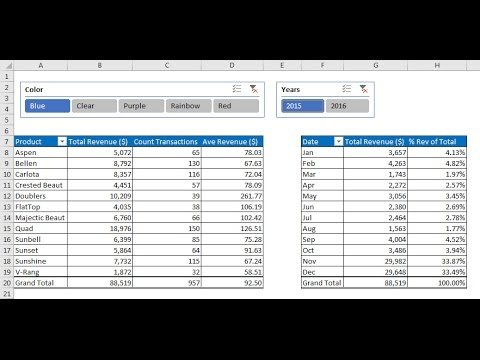# Highline Excel 2016 Class 03: Data Analysis Fundamentals: PivotTables, Power Query & Data Model

In this video learn about the fundamentals of Data Analysis and Business Intelligence in Excel 2016: Sort, Filter, PivotTables, Power Query, Power Pivot Data Model:
1. (00:05) Introduction
2. (01:39) Sort
3. (03:02) Sorting one column
4. (03:23) Sorting multiple columns
5. (09:06) Sorting Mixed Data
6. (10:50) Filter feature
7. (13:22) Filter Drop-down Arrows to see Unique List
8. (15:34) Filter Different Data Types
9. (18:30) Filter to Extract Records
10. (20:54) OR Logical Test (OR Criteria) Discussion
11. (22:52) AND Logical Test (AND Criteria) Discussion
12. (28:23) BETWEEN and NOT Criteria
13. (30:57) PivotTable. Discussion of Crosstabulated tables and PivotTables as “Calculations with Criteria”, both AND Criteria and OR Criteria.
14. (33:05) PivotTable Basics: 1) Drag and Drop Field Names to add criteria to PivotTable, 2) Cross Tabulated Table, 3) Layout Formatting, 4) Number Formatting
16. (40:21) Creating a Custom Style for a PivotTable
17. (44:48) Name PivotTable
18. (46:12) Create PivotTable using “Summarize Values By”, which allows us to change the Aggregate Functions like: SUM, COUNT, AVERAGE.
19. (51:49) Group Dates by Month and Year
20. (54:50) Create PivotTable using “Show Values As” to calculate “% of Column Total”.
21. (56:09) Hide items in Slicer
22. (57:24) Connect Multiple Slicers to Multiple PivotTables.
23. (58:57) Sort in PivotTable.
24. (01:00:11) Create multiple PivotTables with a single click using “Show Report Filter Pages”
25. (01:03:10) Why we need Power Query and Power Pivot Data Model
26. (01:05:21) Introduction to Power Query (Get & Transform)
27. (01:07:04) Power Query Example 1: Clean and Transform Data Table, Create PivotTable Based on Power Query Update, 3) Add new data to table and Refresh to update Query and PivotTable
28. (01:18:25) Power Query to Unpivot a Crosstabulated Table into a Proper Data Set.
29. (01:24:54) Introduction to Power Pivot and the Data Model
30. (01:25:32) Power Query to import multiple Text File tables with over one million records combine them into a single Table. We will use the “From File, From Folder” option.
31. (01:33:20) Load the million records in Power Query into the Power Pivot Data Model.
32. (01:34:42) Add an Excel Table into the Power Pivot Data Model
33. (01:35:30) Update Power Query
34. (01:36:36) Build a relationship between tables in the Power Pivot Data Model.
35. (01:38:08) Build PivotTable from Millions of Records from Two Tables
36. (01:40:34) Add new Text File to Folder and Update PivotTable.
37. (01:41:22) Summary

2697 – 1460931006 – 260172

data analysis excel